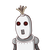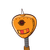# classIn an isosceles triangle, the vertex angle is thrice of either base angle. Find the measuretriangle​

class
In an isosceles triangle, the vertex angle is thrice of either base angle. Find the measure
triangle

### 2 thoughts on “class<br />In an isosceles triangle, the vertex angle is thrice of either base angle. Find the measure<br />triangle<br />​”

1.hahahehhshshshhsbsbshhsss

2.In an isosceles triangle base angles are equal

let each base angle is x°

so, vertex angle is 3x°

sum if the three angles of a triangle is 180°

so,

3x+x+x=180

=> 5x=180

=> x=180/5

=> x=36

so , each of the base angle is 36° and vertex angle is 36×3=108°